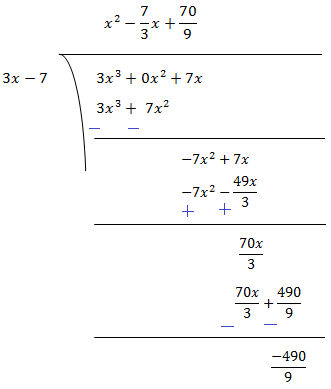# NCERT Solutions for Class 9th: Ch 2 Polynomials Maths (Part -1)

#### NCERT Solutions for Class 9th: Ch 2 Polynomials Maths  (Part -1)

Page No: 32

Exercise 2.1

1. Which of the following expressions are polynomials in one variable and which are not? State reasons for your answer.
(i) 4x2 - 3x + 7
(ii) y2 + √2
(iii) 3√t + t2
(iv) y + 2/y
(v) x10 + y3 + t50

(i) 4x2 - 3x + 7
There is only one variable x with whole number power so this polynomial in one variable.

(ii)  y2 + √2
There is only one variable y with whole number power so this polynomial in one variable.

(iii) 3√2 + t
There is only one variable t but in 3√t power of t is 1/2 which is not a whole number so 3√t + t2 is not a polynomial.

(iv) y + 2/y
There is only one variable but 2/y = 2y-1 so the power is not a whole number so y + 2/y is not a polynomial.

(v) x10 + y3 + t50
There are three variable xy and t and there powers are whole number so this polynomial in three variable.

2. Write the coefficients of x2 in each of the following:
(i) 2 + x2 + x
(ii) 2 - x2 + x3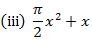(iv) √2x - 1

(i) coefficients of x2 = 1
(ii) coefficients of x2 = -1
(iii) coefficients of x2 = π/2
(iv) coefficients of x2 = 0

3. Give one example each of a binomial of degree 35, and of a monomial of degree 100.

3x35+7 and 4x100

4. Write the degree of each of the following polynomials:
(i) 5x3 + 4x2 + 7x
(ii) 4 – y2
(iii) 5t – √7
(iv) 3

(i) 5x3 has highest power in the given polynomial which power is 3. Therefore, degree of polynomial is 3.

(ii) – y2  has highest power in the given polynomial which power is 2. Therefore, degree of polynomial is 2.

(iii) 5t has highest power in the given polynomial which power is 1. Therefore, degree of polynomial is 1.

(iv) There is no variable in the given polynomial. Therefore, degree of polynomial is 0.

5. Classify the following as linear, quadratic and cubic polynomial:
(i) x2 + x

(ii) x - x3
► Cubic Polynomial

(iii) y + y2 +4

(iv) 1 + x
► Linear Polynomial

(v) 3t
►Linear Polynomial
(vi) r2

(vii) 7x3
► Cubic Polynomial

Page No: 34

Exercise 2.2

1. Find the value of the polynomial at 5x + 4x2 + 3 at

(i) x = 0 (ii) x = - 1 (iii) x = 2

(i) p(x) = 5x + 4x2 + 3
p(0) = 5(0) + 4(0)2 + 3
= 3

(ii) p(x) = 5x + 4x2 + 3
p(-1) = 5(-1) + 4(-1)2 + 3
= -5 + 4(1) + 3 = 2

(iii) p(x) = 5x + 4x2 + 3
p(2) = 5(2) + 4(2)2 + 3
= 10 + 16 + 3 = 29

2. Find p(0), p(1) and p(2) for each of the following polynomials:
(i) p(y) = y2 - y + 1
(ii) p(t) = 2 + t + 2t2 - t3
(iii) p(x) = x3
(iv) p(x) = (x - 1) (x + 1)

(i) p(y) = y2 - y + 1
p(0) = (0)2 - (0) + 1 = 1
p(1) = (1)2 - (1) + 1 = 1
p(2) = (2)2 - (2) + 1 = 3

(ii) p(t) = 2 + t + 2t2 - t3
p(0) = 2 + 0 + 2 (0)2 - (0)3 = 2
p(1) = 2 + (1) + 2(1)2 - (1)3
= 2 + 1 + 2 - 1 = 4
p(2) = 2 + 2 + 2(2)2 - (2)3
= 2 + 2 + 8 - 8 = 4

(iii) p(x) = x3
p(0) = (0)3 = 0
p(1) = (1)3 = 1
p(2) = (2)3 = 8

(iv) p(x) = (x - 1) (x + 1)
p(0) = (0 - 1) (0 + 1) = (- 1) (1) = - 1
p(1) = (1 - 1) (1 + 1) = 0 (2) = 0
p(2) = (2 - 1 ) (2 + 1) = 1(3) = 3

Page No: 35

3. Verify whether the following are zeroes of the polynomial, indicated against them.
(i) p(x) = 3x + 1, x = -1/3
(ii)  p(x) = 5x - π, x = 4/5
(iii) p(x) = x2 - 1, x = 1, -1
(iv) p(x) = (x + 1) (x - 2), x = -1, 2
(v) p(x) = x2 , x = 0(viii) p(x) = 2x + 1, x = 1/2

(i) If x = -1/3 is a zero of polynomial p(x) = 3x + 1 then p(-1/3) should be 0.
At, p(-1/3) = 3(-1/3) + 1 = -1 + 1 = 0
Therefore, x = -1/3 is a zero of polynomial p(x) = 3x + 1.

(ii) If x = 4/5 is a zero of polynomial p(x) = 5x - π then p(4/5) should be 0.
At, p(4/5) = 5(4/5) - π = 4 - π
Therefore, x = 4/5 is not a zero of given polynomial p(x) = 5x - π.

(iii) If x = 1 and x = -1 are zeroes of polynomial p(x) = x2 - 1, then p(1) and p(-1) should be 0.
At, p(1) = (1)2 - 1 = 0 and
At, p(-1) = (-1)2 - 1 = 0
Hence, x = 1 and -1 are zeroes of the polynomial  p(x) = x2 - 1.

(iv) If x = -1 and x = 2 are zeroes of polynomial p(x) = (x +1) (x - 2), then p( - 1) and (2)should be 0.
At, p(-1) = (-1 + 1) (-1 - 2) = 0 (-3) = 0, and
At, p(2) = (2 + 1) (2 - 2) = 3 (0) = 0
Therefore, x = -1 and x = 2 are zeroes of the polynomial p(x) = (x +1) (x - 2).

(v) If x = 0 is a zero of polynomial p(x) = x2, then p(0) should be zero.
Here, p(0) = (0)2 = 0
Hence, x = 0 is a zero of the polynomial p(x) = x2.(viii)  If x = 1/2 is a zero of polynomial p(x) = 2x + 1 then p(1/2) should be 0.
At, p(1/2) = 2(1/2) + 1 = 1 + 1 = 2
Therefore, x = 1/2 is not a zero of given polynomial p(x) = 2x + 1.

4. Find the zero of the polynomial in each of the following cases:
(i) p(x) = x + 5
(ii) p(x) = x - 5
(iii) p(x) = 2x + 5
(iv) p(x) = 3x - 2
(v) p(x) = 3x
(vi) p(x) = axa ≠ 0
(vii) p(x) = cx + d, c ≠ 0, c, are real numbers.

(i) p(x) = x + 5
p(x) = 0
x + 5 = 0
x = -5
Therefore, x = -5 is a zero of polynomial p(x) = x + 5 .

(ii) p(x) = x - 5
p(x) = 0
x - 5 = 0
x = 5
Therefore, x = 5 is a zero of polynomial p(x) = x - 5.

(iii) p(x) = 2x + 5
p(x) = 0
2x + 5 = 0
2x = -5
x = -5/2
Therefore, x = -5/2 is a zero of polynomial p(x) = 2x + 5.

(iv) p(x) = 3x - 2
p(x) = 0
3x - 2 = 0
x = 2/3
Therefore, x = 2/3 is a zero of polynomial p(x) = 3x - 2.

(v) p(x) = 3x
p(x) = 0
3x = 0
x = 0
Therefore, x = 0 is a zero of polynomial p(x) = 3x.

(vi) p(x) = ax
p(x) = 0
ax = 0
= 0
Therefore, x = 0 is a zero of polynomial p(x) = ax.

(vii) p(x) = cx + d
p(x) = 0
cx + d = 0
x = -d/c
Therefore, x = -d/c is a zero of polynomial p(x) = cx + d.

Page No: 40

Exercises 2.3

1. Find the remainder when x3 + 3x2 + 3x + 1 is divided by
(i) x + 1
(ii) x - 1/2
(iii) x
(iv) x + π
(v) 5 + 2x

(i) x + 1
By long division,Therefore, the remainder is 0.

(ii) x - 1/2
By long division,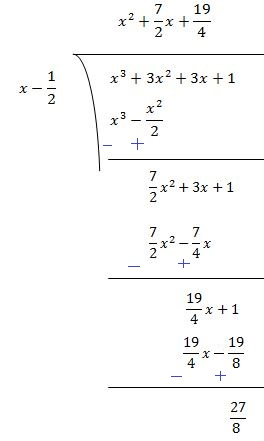Therefore, the remainder is 27/8.

(iii) x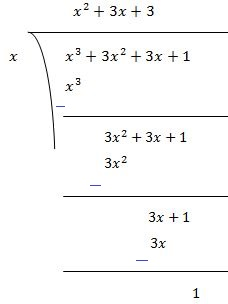Therefore, the remainder is 1.

(iv) x + π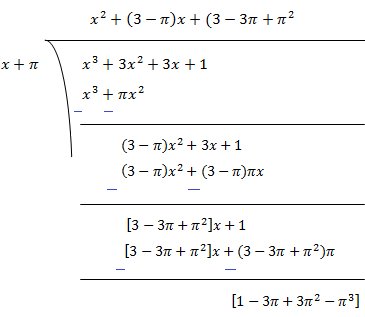Therefore, the remainder is [1 - 3π + 3π2 - π3].

(v) 5 + 2xTherefore, the remainder is -27/8.

2. Find the remainder when x3 - ax2 + 6x - a is divided by x - a.